StatLect

# Maximum likelihood - Algorithm

In the lecture entitled Maximum likelihood we have explained that the maximum likelihood estimatorof a parameteris obtained as a solution of a maximization problem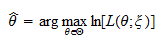where:

1.is the parameter space;

2.is the observed data (the sample);

3.is the likelihood of the sample, which depends on the parameter;

4. theoperator gives the parameter for which the log-likelihoodattains its maximum value.

In several interesting cases, the above maximization problem has an analytical solution. In other words, it is possible to writeexplicitly as a function of the data (see, e.g., maximum likelihood estimation of the parameter of the exponential distribution). However, there are also many cases in which the above problem has no explicit solution. In these cases, it is necessary to resort to numerical algorithms for the maximization of the log-likelihood.

How do these algorithms work?

They are based on two distinct computer programs. The first program is a function (call it FUN) that takes as arguments a value for the parameter vectorand the data, and returns as output the value taken by the log-likelihood. This is illustrated by the following diagram.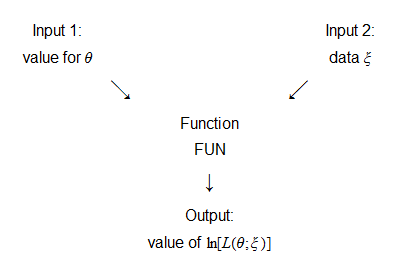The second program is a routine that invokes the function FUN several times. Each time, a different guess ofis provided as an input, the function FUN returns as output the value of the log-likelihood corresponding to that guess, and this output value is stored in the computer memory. When the routine finds a "good guess", according to a pre-specified criterion, execution is stopped and the guess is used as an approximate solution of the maximization problem. This is illustrated by the following diagram.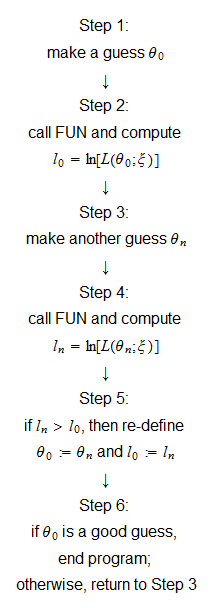There are whole branches of mathematics that are uniquely concerned with devising algorithms capable of performing the above tasks in an effective and efficient manner. Basically, these algorithms consist in a couple of rules: one for generating new guesses of the solution (Step 3), and one for deciding when a guess is good enough (Step 6).

Often, the properties of the log-likelihood function to be maximized, together with the properties of the algorithm, guarantee that the proposed solution converges to the true solution, in the sense that the distance between the true solution and the proposed solution can be made as small as desired by letting the routine perform a sufficiently high number of iterations. However, this kind of convergence, called numerical convergence, cannot always be guaranteed on a theoretical basis, for example, because the properties of the log-likelihood function are difficult to study, or are not sufficient to prove numerical convergence, given the chosen algorithm.

When there is no theoretical guarantee that numerical convergence can be achieved, a heuristic approach is usually followed: a numerical optimization algorithm is run several times, with different, and possibly random, starting values for the parameters (i.e., different initial guesses in Step 1); if all runs of the algorithm (or a majority of them) lead to the same proposed solution (up to small numerical differences), then this is considered as evidence that the proposed solution is a good approximation of the true solution. This approach is called multiple starts, or multi-start, approach (see, e.g., Schoen 1991).

Entering into the mathematical details of numerical optimization would lead us too far astray. For concreteness, the next sections address in a qualitative fashion some practical issues that anyone dealing with maximum likelihood problems should be aware of. After discussing these issues, we will propose some examples.## More details

### Minimizers and maximizers

Commonly available algorithms for numerical optimization usually perform minimization of a function by default. The maximum likelihood problem can be readily adapted to be solved by these algorithms. It suffices to note that finding the maximum of a function is the same as finding the minimum of that function with its signed changed. In other words, solvingis the same as solving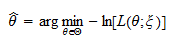### Constrained vs unconstrained optimization

Let the parameterbe a-dimensional vector. If the parameter spaceis the whole set of-dimensional real vectors, i.e.,then an algorithm for unconstrained optimization can be used. This means that there are no constraints on the parameter space and the algorithm will search the whole spacefor a solution. Otherwise, if the parameter space is smaller than the set of-dimensional real vectors, i.e.,wheredenotes strict inclusion, then an algorithm for constrained optimization can be used. This simply means that the algorithm can no longer search the whole spacefor a solution, but it must restrict itself to the subset.

Algorithms for constrained optimization usually require that the parameter spacebe specified in terms of equality or inequality constraints on the entries of.

Example If the parameter is-dimensional and its second entry cannot be negative, the parameter space is specified as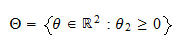whereis the second entry of the parameter. Another example would be the set of-dimensional vectors such that the sum of their entries in less than or equal to: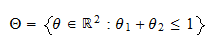Note that asymptotic normality of the maximum likelihood estimator is based on the existence of the derivatives of the log-likelihood function at(the true parameter value). Furthermore, estimation of the asymptotic covariance matrix requires computation of the derivatives of the log-likelihood function at(see the lecture entitled Maximum likelihood - Covariance matrix estimation). Because the derivatives of a function defined on a given set are well-defined only at points that belong to the interior of that set, it follows that the standard results based on asymptotic normality cannot be used whenorare on the boundaries of, that is, when the constraints are binding. There are techniques to derive the asymptotic distribution of the maximum likelihood estimator when the constraints are binding, but these techniques are extremely complex and their applicability is often limited (see, e.g., Newey and McFadden - 1994).

Furthermore, most software packages usually include robust and well-tested algorithms for unconstrained optimization, but reliable routines for constrained optimization can be harder to find or are difficult to use efficiently.

For the reasons explained above, efforts are usually made to avoid constrained optimization problems as much as possible. For instance, several constrained optimization problems can be re-parametrized as unconstrained problems. We give some examples of how this can be accomplished.

Example Suppose a parameterneeds to be strictly positive, i.e.,. We can re-parametrize it asso that there are no constraints on the new parameter, because the original constraint is always respected for.

Example Suppose a parameterneeds to be inside the unit interval, i.e.,. We can re-parametrize it asso that there are no constraints on the new parameter, because the original constraint is always respected for.

Example Suppose two parameters need to satisfy the constraint. We can substitutein the log-likelihood function and reduce the dimensionality of the problem by dropping the parameter.

Also, a constrained optimization problem is sometimes converted into an unconstrained one by using penalties. This is done as follows: instead of solving the constrained problema solution is sought for the unconstrained modified problem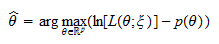whereis a penalty function defined as follows: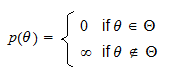In other words, the optimization algorithm is allowed to search the whole spacefor a solution, but when the algorithm proposes a guess that falls outside the parameter space, the function returns a value of, so that the guess will never be selected as a solution. Because the infinite penaltyis discontinuous and non-differentiable, it is often replaced by penalties that are continuous and differentiable and that are numerically very close to it (see, e.g., Griva, Nash and Sofer 2009). This can lead to significant gains in terms of efficiency and speed of the optimization. Keep in mind, however, that modern optimization software is often capable of dealing with infinite penalties.

### Choice of a specific algorithm

Thousands of optimization algorithms have been proposed in the literature (see, e.g., Wikipedia's article on Optimization techniques). The main differences between these algorithms are: whether or not they require the computation of the derivatives of the function to be optimized, whether or not they are able to guarantee numerical convergence, whether or not they can deal with non-smooth (i.e., non-continuous or non-differentiable) functions.

Unless you are an expert in the field, it is generally not a good idea to decide yourself which of these algorithms to use and to write a computer routine from scratch to implement it. In most cases, your best option is to use the optimization routines that are already built in the statistical software you are using to carry out maximum likelihood estimation. Typically, the choice will be quite limited, so you can try what seems to work best for you. For example, in MATLAB you have basically two built-in algorithms, one called `fminsearch`, that does not require the computation of derivatives, and one called `fminunc`, that does require it. The first one tends to be slow, but is quite robust and can deal also with ill-behaved or discontinuous functions, while the second one is much faster, but cannot properly deal with non-smooth functions. Whatever your choice, remember that the multi-start approach (see above) provides tremendous value, so always re-run your optimizations several times, with different (and possibly random) starting points, in order to check that the proposed solution is stable.

### Derivatives based algorithms

Several algorithms require as input first- and second-order derivatives of the log-likelihood function, and use these derivatives to form new guesses of the parameter value. Depending on the algorithm, these derivatives can either be provided by the user, in the form of a function that computes the values of the derivatives at each parameter value, or are computed directly by the optimization algorithm, by using numerical differentiation techniques. Remember that numerical differentiation techniques tend to be unstable, so, if the derivatives of the log-likelihood function can be computed analytically, it is preferable to provide them to the optimization algorithm.

### Stopping criteria

As we have seen, a numerical optimization algorithm keeps proposing new guesses of the solution until it finds a good guess, according to some pre-specified criterion (see Step 6 in the diagram above). What criteria are usually adopted to decide whether a guess is good enough? There are several common criteria, and they are often used in conjunction. Some of these criteria are briefly described below.

• Termination tolerance on the log-likelihood. The algorithm stops when the new guesses produce only minimal increments of the log-likelihood function, that is, when only negligible improvements of the solution can be achieved by performing new iterations. What increments are to be considered negligible is decided by the user. For example, if the termination tolerance is set toby the user, this means that the algorithm will keep proposing new guesses only if the value of the log-likelihood function increases by at leastwhen the previous guesses are replaced with the new ones.

• Termination tolerance on the parameter. The algorithm stops when the new guesses are almost identical to the previous ones, that is, when performing new iterations changes only minimally the proposed solution. As in the previous case, what changes in the parameter value are to be considered negligible is decided by the user.

• Maximum number of iterations. Because there is often no guarantee that one of the previous stopping criteria will be met after a reasonable number of iterations, optimization algorithms usually also require to specify a maximum number of iterations after which execution will be stopped.

## Examples

An example of how to perform maximum likelihood estimation with MATLAB is provided in the lecture entitled Maximum likelihood - MATLAB example.

## References

Griva, I., Nash, S., and Sofer, A. (2009) Linear and Nonliner Optimization, 2nd Edition, SIAM.

Newey, W. K. and D. McFadden (1994) "Chapter 35: Large sample estimation and hypothesis testing", in Handbook of Econometrics, Elsevier.

Schoen, F. (1991) " Stochastic techniques for global optimization: a survey of recent advances", Journal of Global Optimization, 1, 207-228.

The book

Most of the learning materials found on this website are now available in a traditional textbook format.

Glossary entries
Share